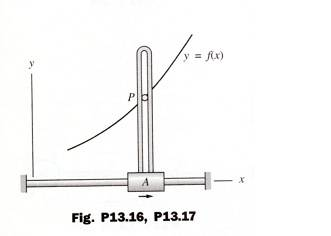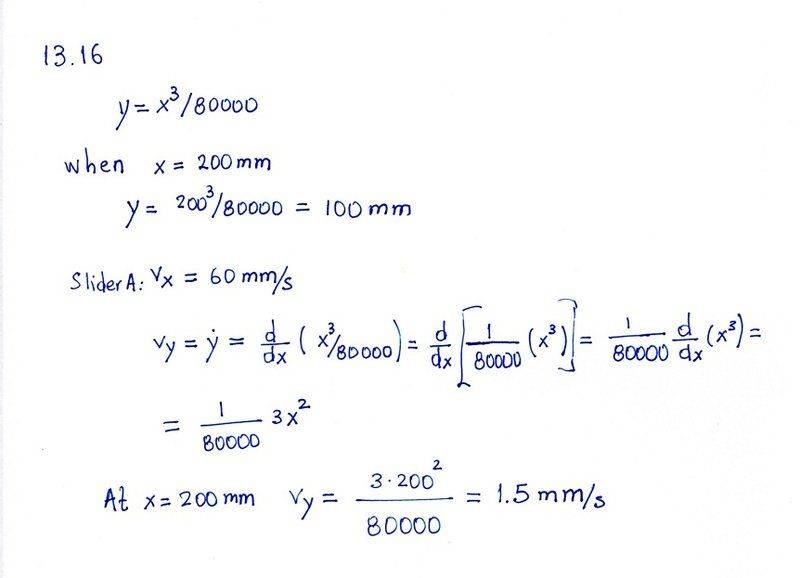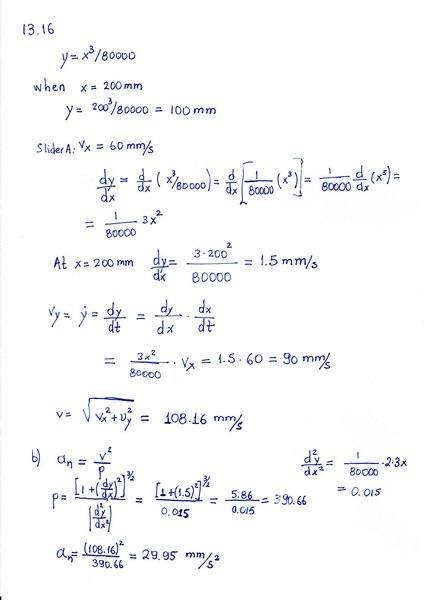Pen P of the flatbed plotter traces the curve - Path Coordinates

Homework Statement

This is Pytels Dynamics 2nd edition problem 13.16

13.16. Pen P of the flatbed plotter traces the curve y=x3/80000, where x and y are
measured in mm. When x=200mm, the speed of slider A is 60 mm/s. For this position, calculate
(a) the speed of P; and (b) the normal component of the acceleration of PThe Attempt at a SolutionIn part (a) I don't get 108.2 mm/s for the speed. what am I doing wrong?

TSny
Homework Helper
Gold Member
##\dot y## is the derivative of ##y## with respect to time. You took the derivative of ##y## with respect to ##x##. Hint: chain rule.

I guess this is correct. Right?TSny
Homework Helper
Gold Member
That look's correct. Nice work.